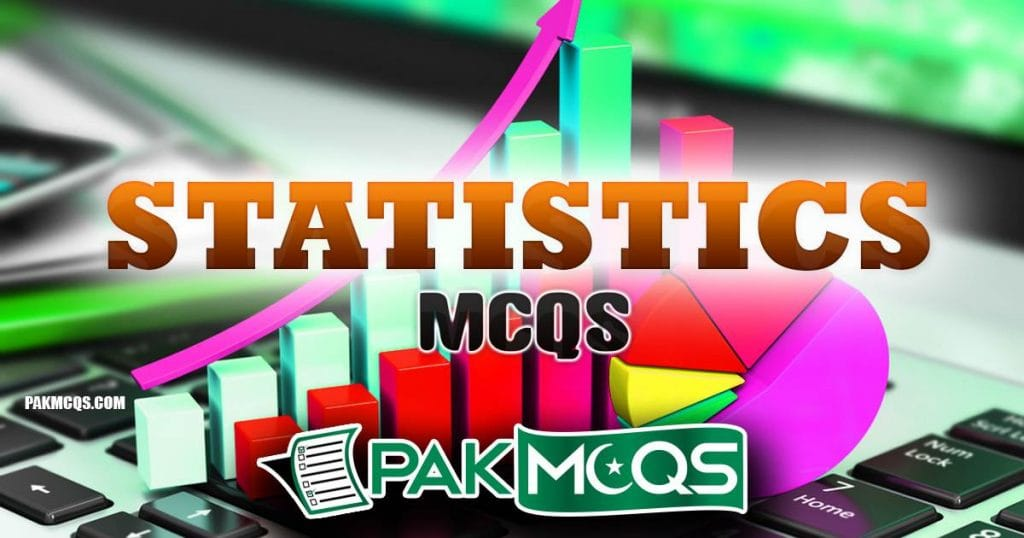Presentation Of Data
Presentation Of Data Mcqs for Statistics
Statistics McqsStatistics Mcqs for the Prepration of FPSC Tests, PSC Tests, NTS Test.  Here you will find Basic statistics mcqs , data, Sample, population, Measure of dispersion, Measure of central tendency, Descriptive Statistics, Inferential Statistics etc.
STATISTICS MCQS
1.Basic Statistics8.Probability
2.Sampling9.Sample Linear Regression
3.Correlation and Regression10.Statistical Inference
4.Binomial And Hypergeometric Probability11.Index Number
5.Measures Of Dispersion12.Normal Distribution
6.Presentation Of Data13.Measures Of Location
7.Chart and Graph14.Hypothesis

IF YOU THINK THAT ABOVE POSTED MCQ IS WRONG.

PLEASE COMMENT BELOW WITH CORRECT ANSWER AND ITS DETAIL EXPLANATION.